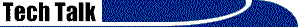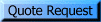# Implied Decimal

 Need to convert COBOL data?That's our business!

Implied decimal simply means there is a decimal point implied at a specified location in a field, but not actually present in the file.  For example, the number 123 with an implied decimal of two digits represents the actual value 1.23  Using implied decimal saves space in the file.  Implied decimal can apply to any kind of numeric field, including a binary, packed, or comp-3 field.

Most PC applications don't understand implied decimal and need a real decimal.  DISC can add a real decimal during the conversion of your file.  If we are writing a COBOL program to convert your data, changing implied decimal to real decimal is not much more work or cost.  If we are doing a simple EBCDIC to ASCII conversion via a translation table, it's not simple to add a real decimal.

Converting implied decimal to real decimal increases the size of the field, and this may cause other complications, such as shifting other fields in the record, and alignment issues with redefined fields.